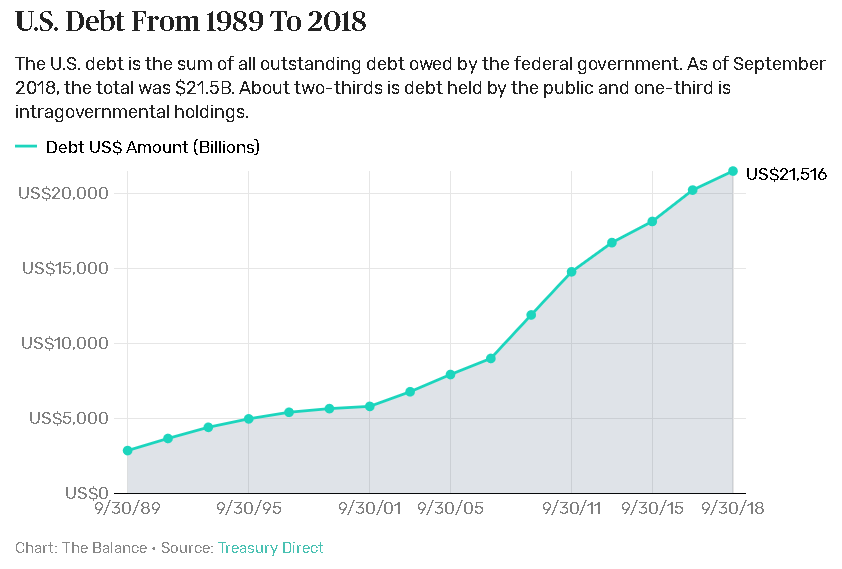# People of united states need help

People of united states need help or their elite will lead them to war and destruction.

The people of united states need friends that relieve them from fear, they are capable of spending to bankruptcy.

Russia, China, and Iran must read the history of the first half of 20th century and notice that

a bankrupt super power is not good for anyone.

FY DoD Base Budget DoD OCO Support Base Support OCO Total Spending
2003 $364.9$72.5     $437.4 2004$376.5 $91.1$467.6
2005 $400.1$78.8     $478.9 2006$410.6 $124.0$109.7   $644.3 2007$431.5 $169.4$120.6   $721.5 2008$479.0 $186.9$127.0   $792.9 2009$513.2 $153.1$149.4   $815.7 2010$527.2 $163.1$160.3 $0.3$851.6
2011 $528.2$158.8 $167.4$0.7 $855.1 2012$530.4 $115.1$159.3 $11.5$816.3
2013 $495.5$82.1 $157.8$11.0 $746.4 2014$496.3 $85.2$165.4 $6.7$753.6
2015 $496.1$64.2 $165.6$10.5 $736.4 2016$521.7 $58.9$171.9 $15.1$767.6
2017  $523.2$82.5 $177.1$35.1 $818.9 2018 Actual$574.5 $88.1$181.8 $46.4$890.8
2019 Estimated $657.0$69.0 $214.4$26.1 $956.5 2020 Budget$576.0 $174.0$212.9 $26.1$989.0

https://www.thebalance.com/u-s-military-budget-components-challenges-growth-3306320https://www.thebalance.com/the-u-s-debt-and-how-it-got-so-big-3305778